# Articles & photos

Buell specification by model

28-5-2020 - Here is a list of Buell Motorcycles Produced and there specifications

RW 750 = 2

Buell S1 White Lightning = 2,210

Buell XB9SX Lightning City X = 9,632

Buell RR1000 Battletwin = 51

Buell M2L Cyclone Low = 1,761

Buell XB12Ss Lightning Long = 7,013

Buell RR1200 Battletwin = 59

Buell X1 Lightning = 11,228

Buell XBRR = 56

Buell RS1200 Westwind = 208

Buell X1M Lightning Millennium = 661

Buell XB12X Ulysses = 7,432

Buell RSS1200 Westwind = 98

Buell Blast = 22,785

Buell XB12STT Lightning Super TT = 1,755

Buell S2 Thunderbolt = 1,694

Buell XB9R Firebolt = 7,484

Buell 1125R = 5,836

Buell S2T Thunderbolt = 429

Buell XB9S Lightning = 4,332

Buell XB12XT Ulysses = 1,911

Buell S1 Lightning = 4,680

Buell XB9SL Lightning Low = 1,513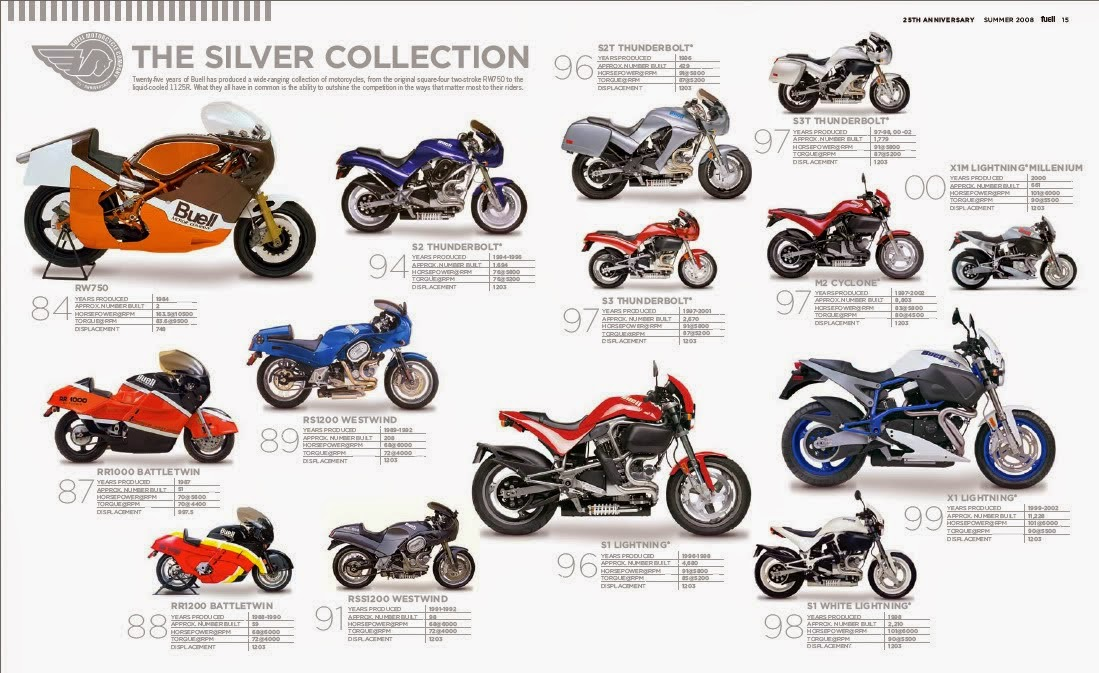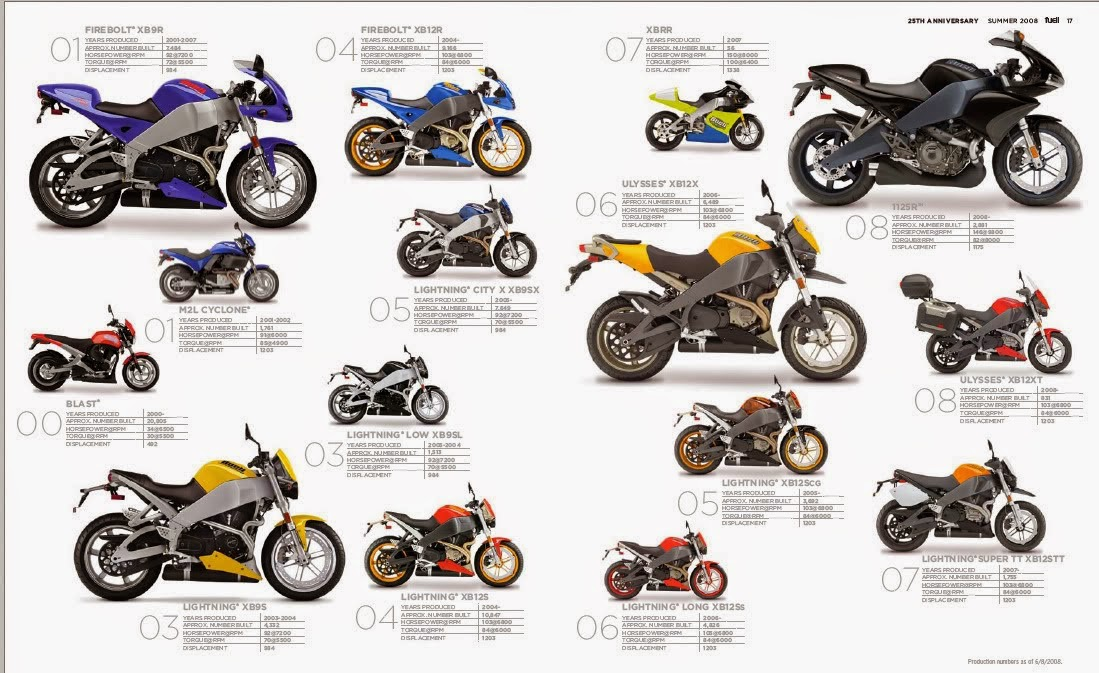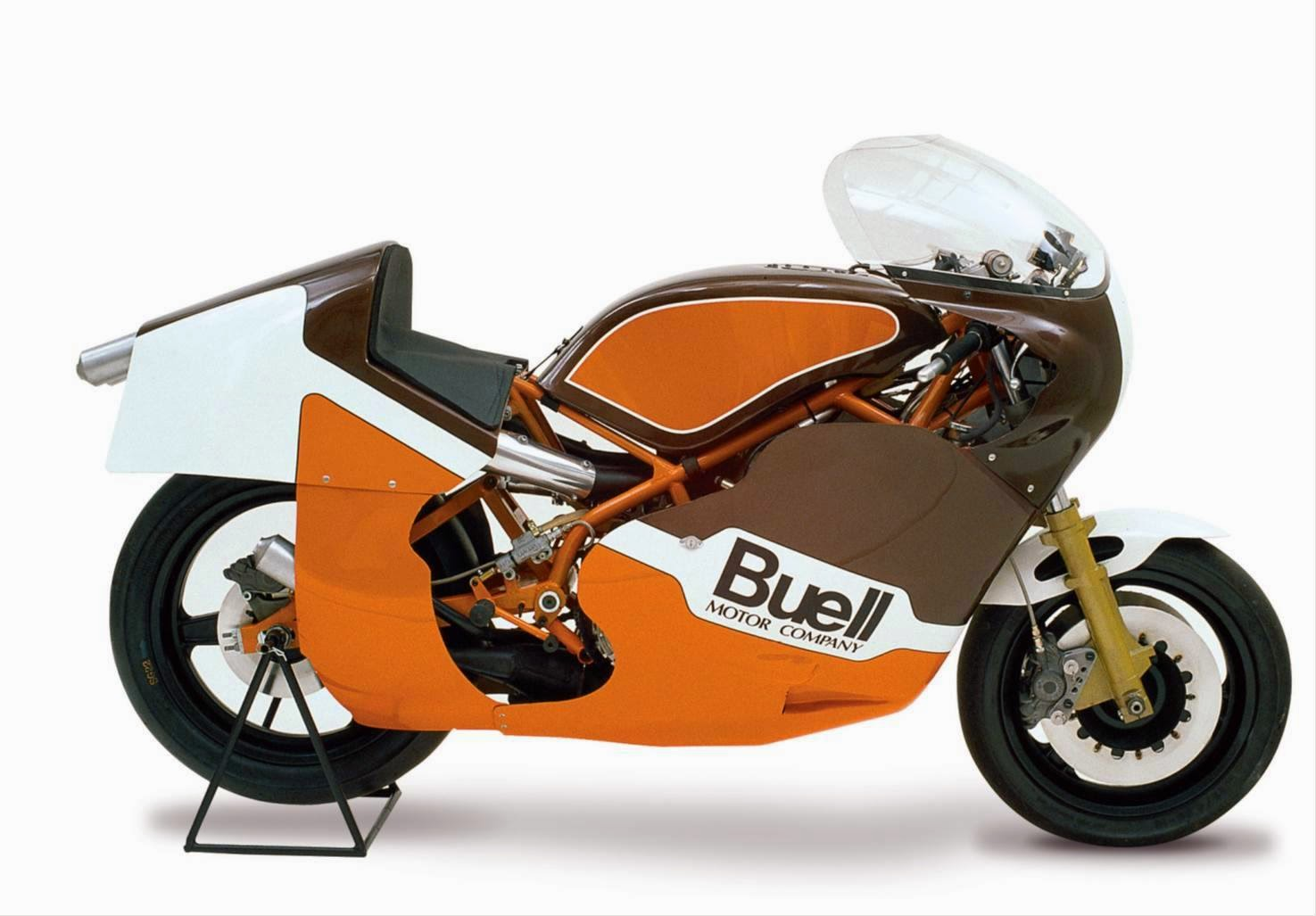### BUELL RW 750

PRODUCED 1983

APPROX. NUMBER BUILT - 2
Displacement: 748cc

163.5HP @10500 RPM
Torque: 83.6 ft.lbs @ 9,500 rpm

### BUELL RR 1000 BATTLETWIN

MODEL YEARS PRODUCED 1987
APPROX. NUMBER BUILT - 51
Displacement: 997.5cc
70HP@5600RPM
Torque: 70 ft.lbs @ 4,400 rpm### BUELL RR 1200 BATTLETWIN

MODEL YEARS PRODUCED 1988-1990
APPROX. NUMBER BUILT - 59

1988 = 9

1989 = 34

1990 = 16
Displacement: 1203cc
68HP@6000RPM
Torque: 72 ft.lbs @ 4,000 rpm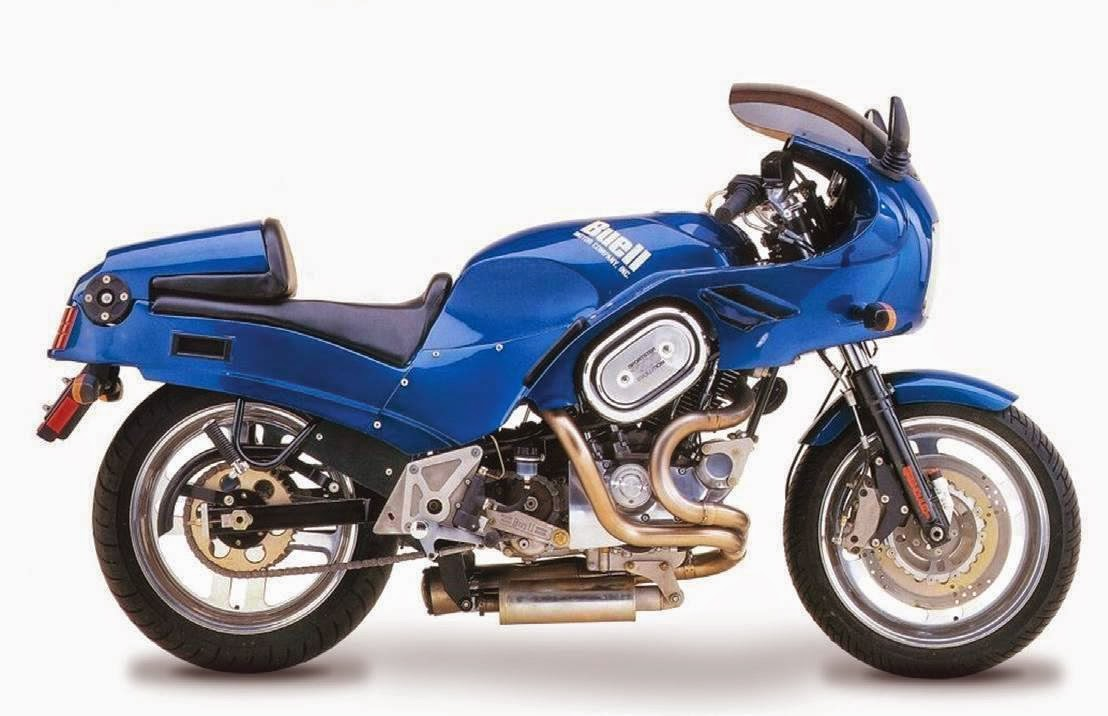### BUELL RS 1200 WESTWIND

MODEL YEARS PRODUCED 1989-1992

APPROX. NUMBER BUILT - 208

1989 = 7

1990 = 94

1991 = 78

1992 = 29

Displacement: 1203cc

68HP@6000RPM

Torque: 72 ft.lbs @ 4,000 rpm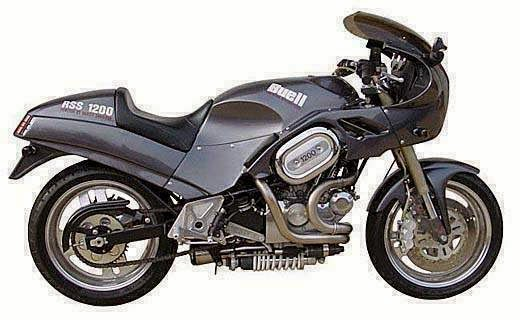### BUELL RSS 1200 WESTWIND

MODEL YEARS PRODUCED 1991-1992

APPROX. NUMBER BUILT -98

1991 = 35

1992 = 63

Displacement: 1203cc

Horsepower: 68 @ 6,000 rpm

Torque: 72 ft.lbs @ 4,000 rpm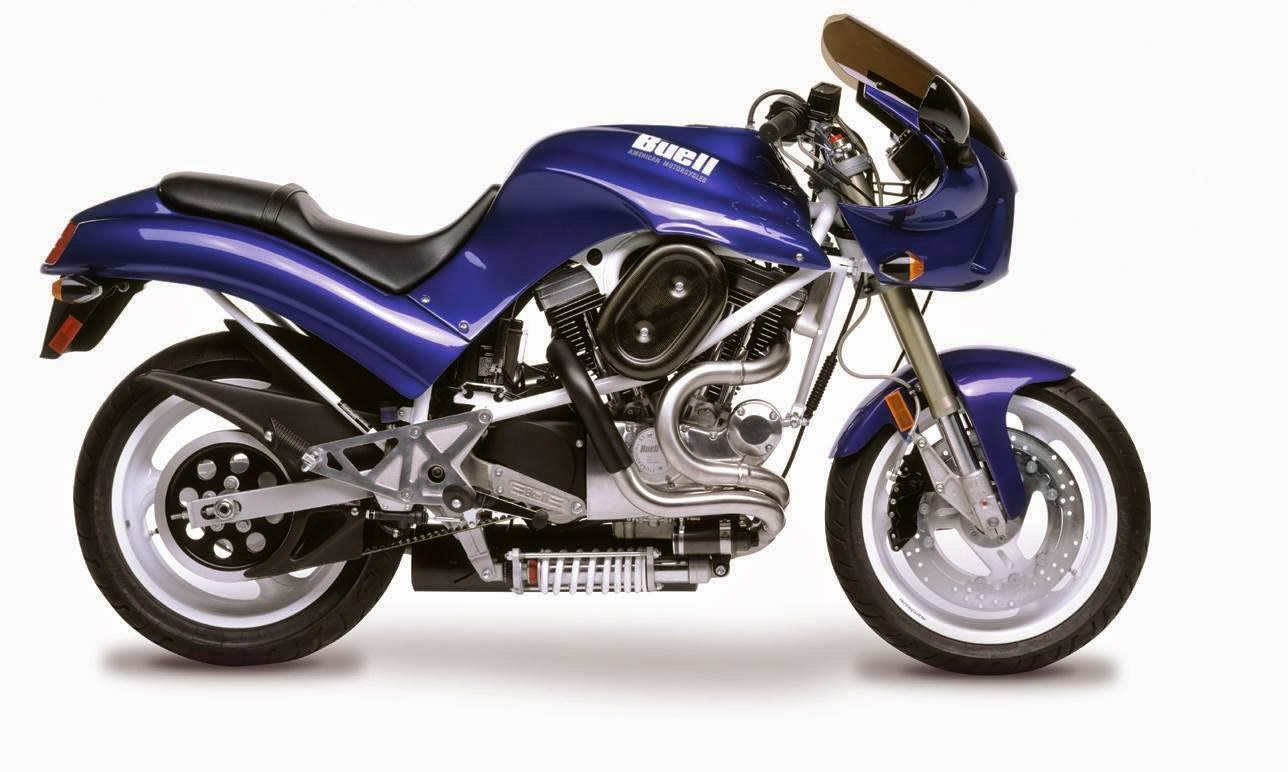### BUELL S2 THUNDERBOLT

MODEL YEARS PRODUCED 1995-1996

APPROX. NUMBER BUILT - 1,694

1995 = 1,471
1996 = 223
Displacement: 1203cc
76HP@5250RPM
Torque: 78 ft.lbs @ 4,500 rpm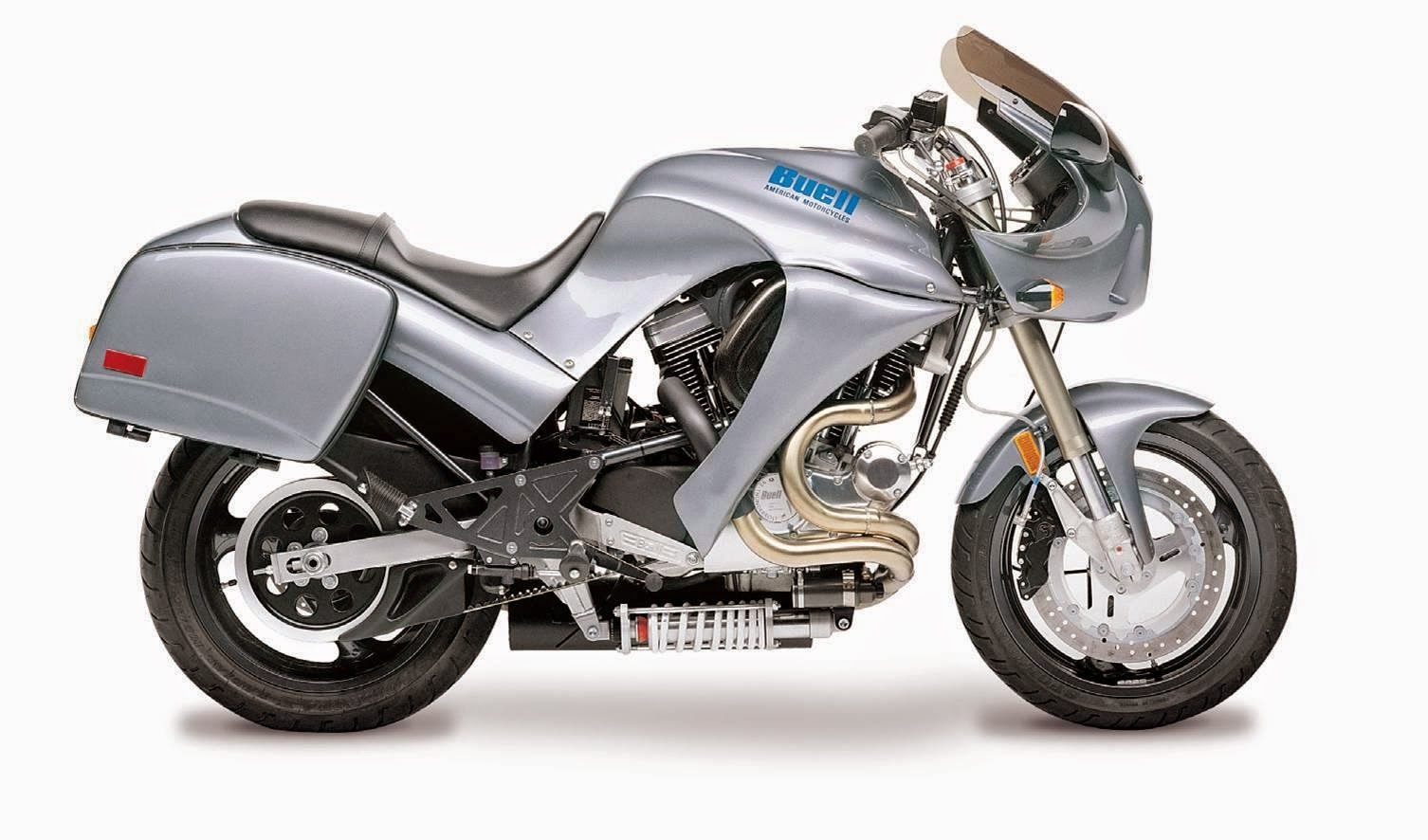### BUELL S2T THUNDERBOLT

MODEL YEARS PRODUCED 1996

APPROX. NUMBER BUILT - 429
Displacement: 1203cc
76HP@5250RPM
Torque: 78 ft.lbs @ 4,500 rpm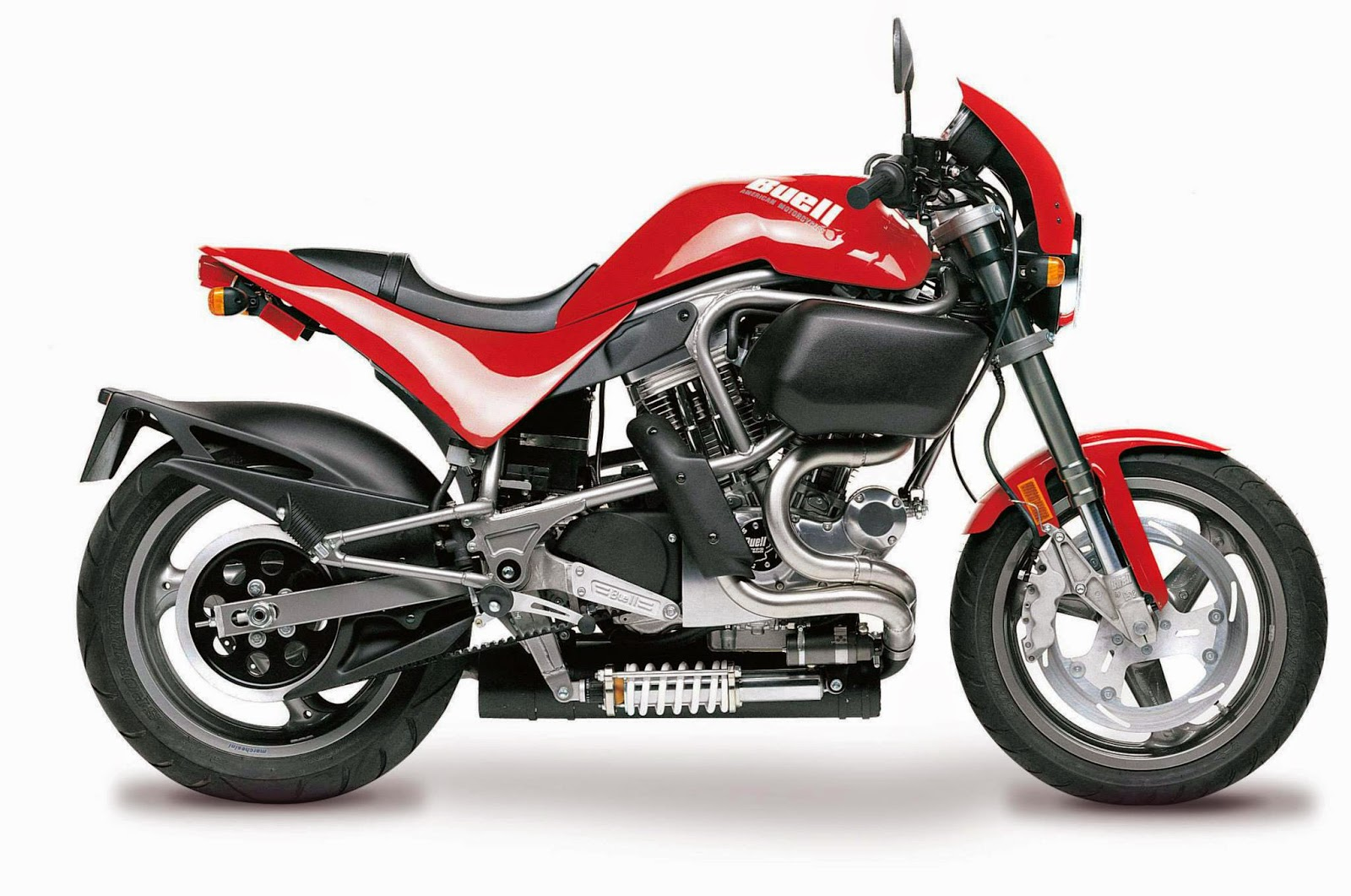### BUELL S1 LIGHTNING

MODEL YEARS PRODUCED 1996-1998

APPROX. NUMBER BUILT 4,680
1996 = 1,453
1997 = 1,767
1998 = 1,460
Displacement: 1203cc

91HP@5800RPM
Torque: 85 ft.lbs @ 5,200 rpm### BUELL S3T THUNDERBOLT

MODEL YEARS PRODUCED 97-98, 00-02

APPROX. NUMBER BUILT 1,779

1997 = 384

1998 = 630

1999 = NIL

2000 = 349

2001 = 164

2002 = 252

Displacement: 1203cc

91HP@5800RPM
Torque: 87 ft.lbs @ 5,200 rpm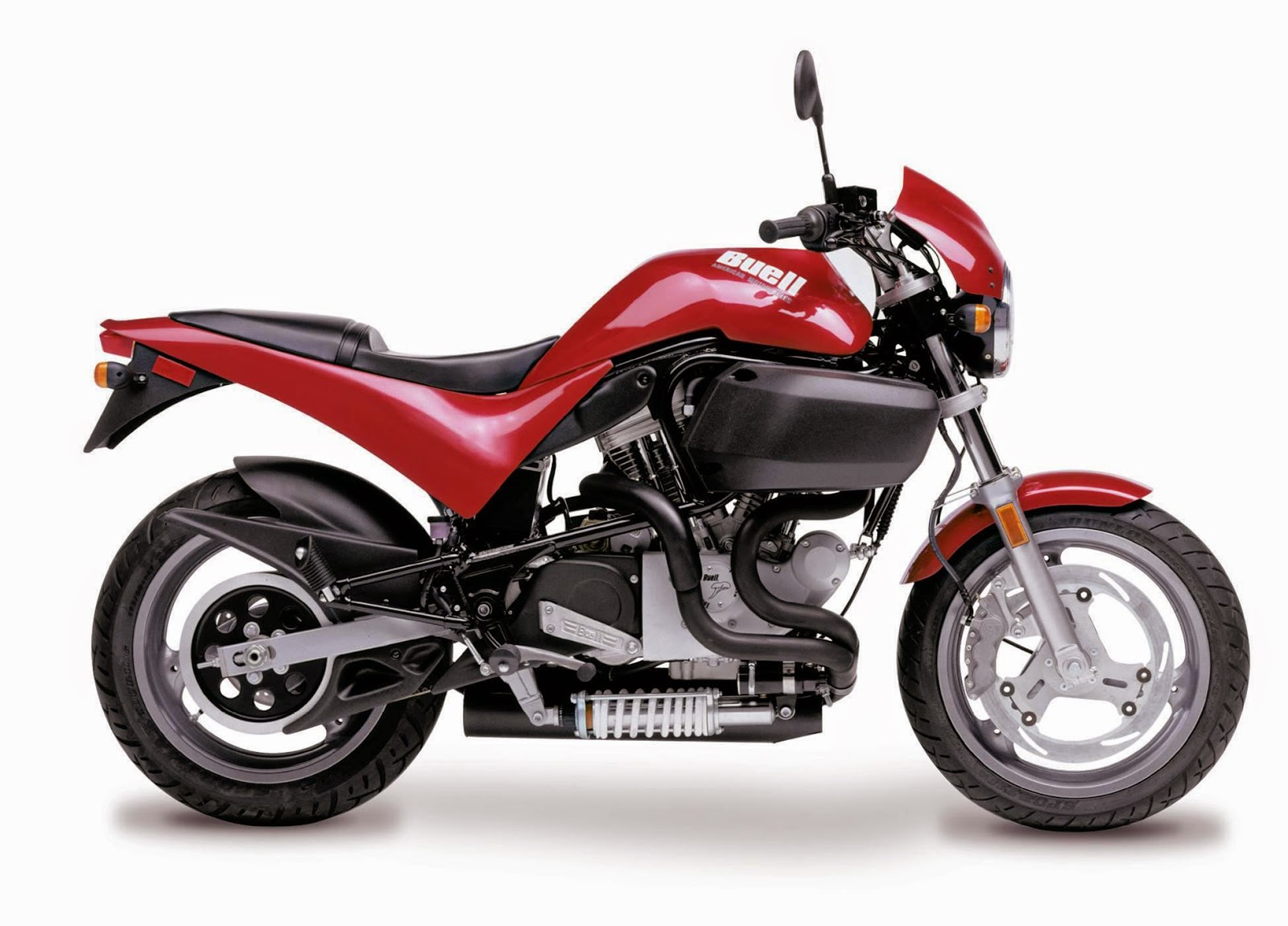### BUELL M2 CYCLONE

MODEL YEARS PRODUCED 1997-2002

APPROX. NUMBER BUILT - 8,803

1997 = 1,138

1998 = 943

1999 = 2,039

2000 = 3,096

2001 = 892

2002 = 695

Displacement: 1203cc

83HP@5800RPM
Torque: 80 ft.lbs @ 4,500 rpm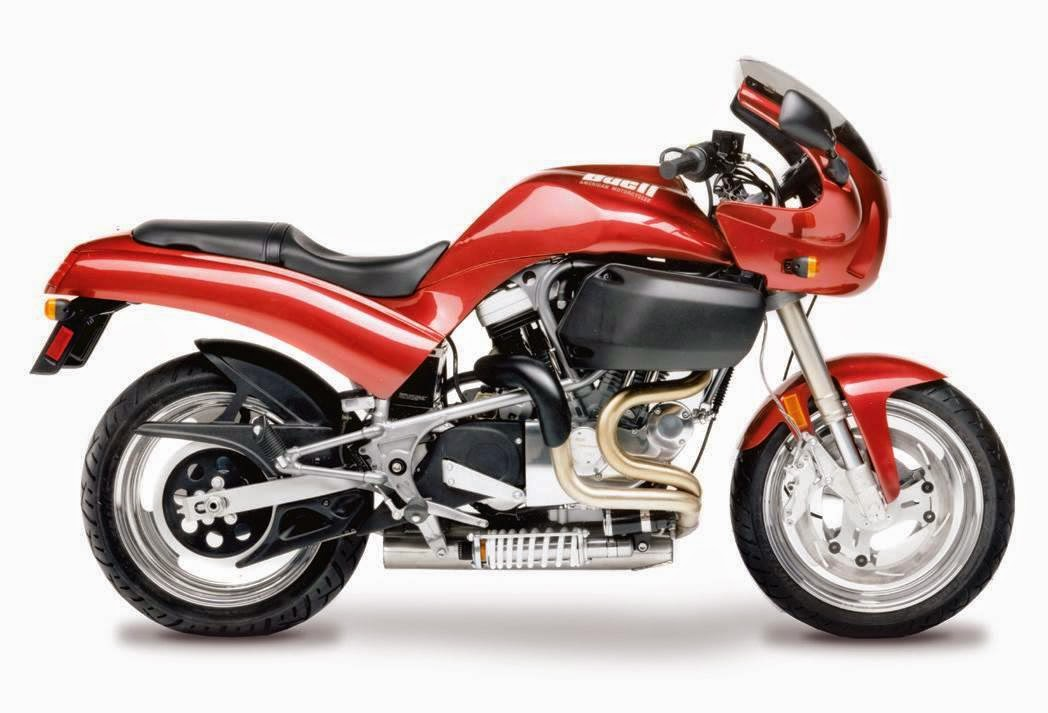### BUELL S3 THUNDERBOLT

MODEL YEARS PRODUCED 1997-2002

APPROX. NUMBER BUILT - 2,672

1997 = 673

1998 = 607

1999 = 956

2000 = 352

2001 = 82

2002 = 2

Displacement: 1203cc

91HP@5800RPM
Torque: 87 ft.lbs @ 5,200 rpm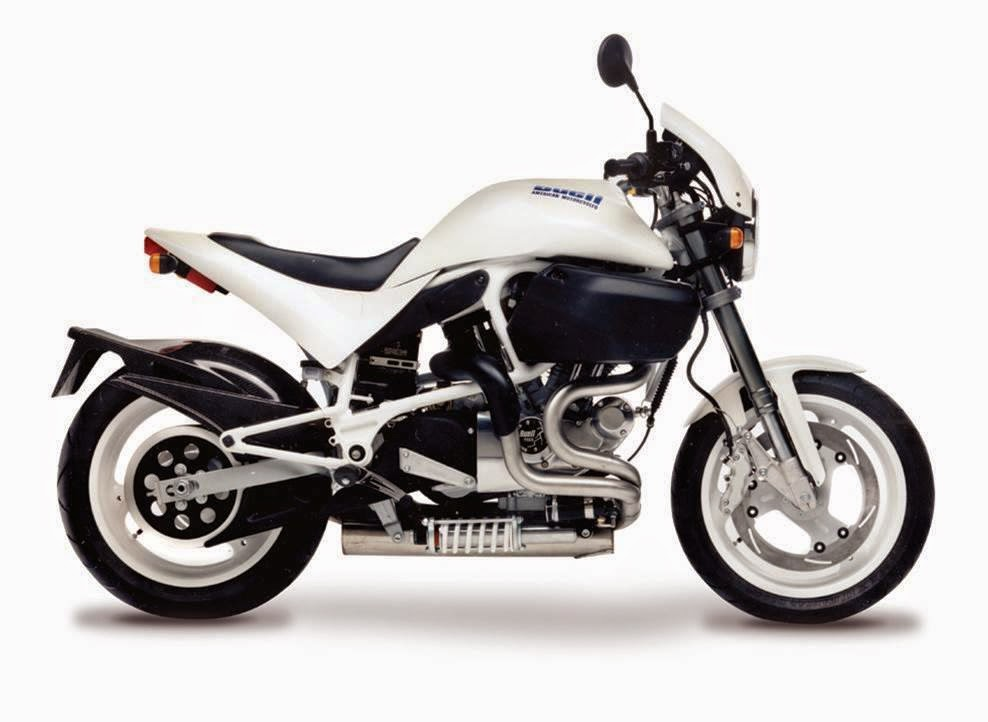### BUELL S1 WHITE LIGHTNING

MODEL YEARS PRODUCED 1998

APPROX. NUMBER BUILT - 2,210
Displacement: 1203cc

101HP@6000RPM
Torque: 90 ft.lbs @ 5.500 rpm

- 1258 Ice White Pearl
- 378 Carbon Black
- 295 Molton Orange
- 138 Billet Metallic

BUELL S1 WHITE LIGHTNING RAINBOW COLLECTION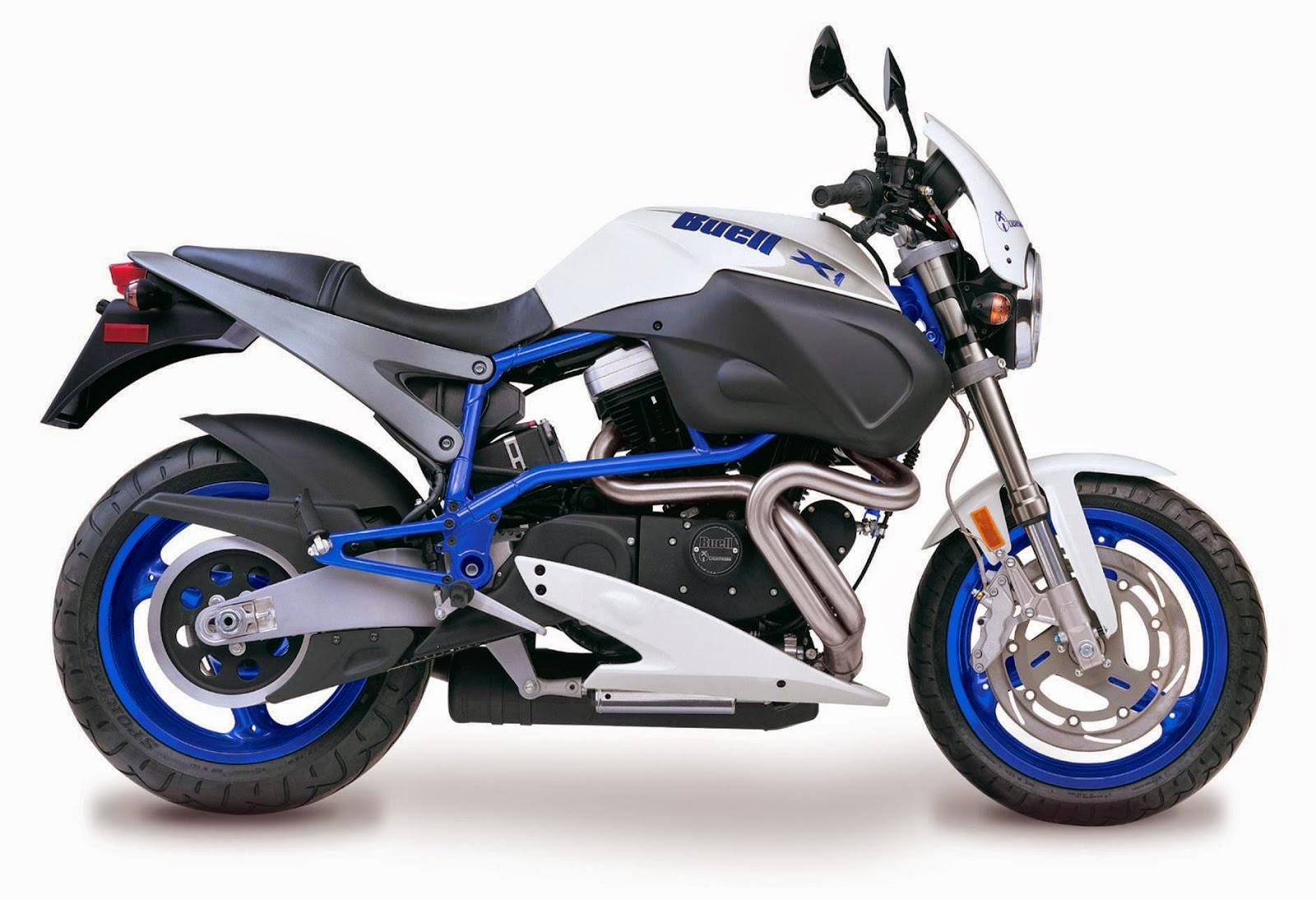### BUELL X1 LIGHTNING

MODEL YEARS PRODUCED 1999-2002

APPROX. NUMBER BUILT - 11,228

(This figure includes limited edition variants such as the ‘Red Stripe’ etc.)

1999 = 3,544

2000 = 3,576

2001 = 2,458

2002 = 1,650

Displacement: 1203cc

101HP@6000RPM
Torque: 90 ft.lbs @ 5,500 rpm
X1 Viper = 800
X1 Millenium = 800
X1 White Lightning = 749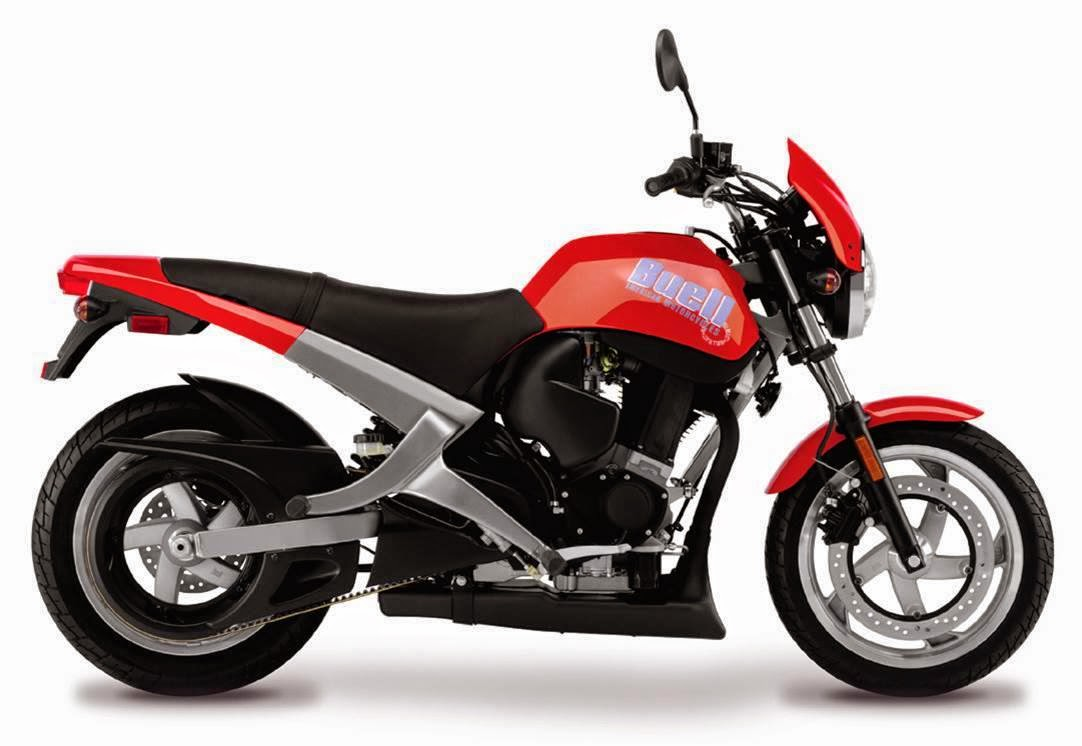### BUELL BLAST

MODEL YEARS PRODUCED 2000-2010

APPROX. NUMBER BUILT - 22,785

2000 = 2,280

2001 = 4,751

2002 = 3,787

2003 = 2,921

2004 = 1,245

2005 = 1,620

2006 = 1,437

2007 = 1,488

2008 = 1,360

2009 = 1,349

2010 = 547

Displacement: 492cc

Horsepower: 34 @ 6,500 rpm

Torque: 30 ft.lbs @ 5,500 rpm

### BUELL XB9R FIREBOLT

Model years: 2003 to 2007

Number produced: 7,484

2003 = 6,297

2004 = 153

2005 = 440

2006 = 226

2007 = 368

Displacement: 984cc

Horsepower: 92 @ 7,500 rpm

Torque: 68 ft.lbs @ 5,500 rpm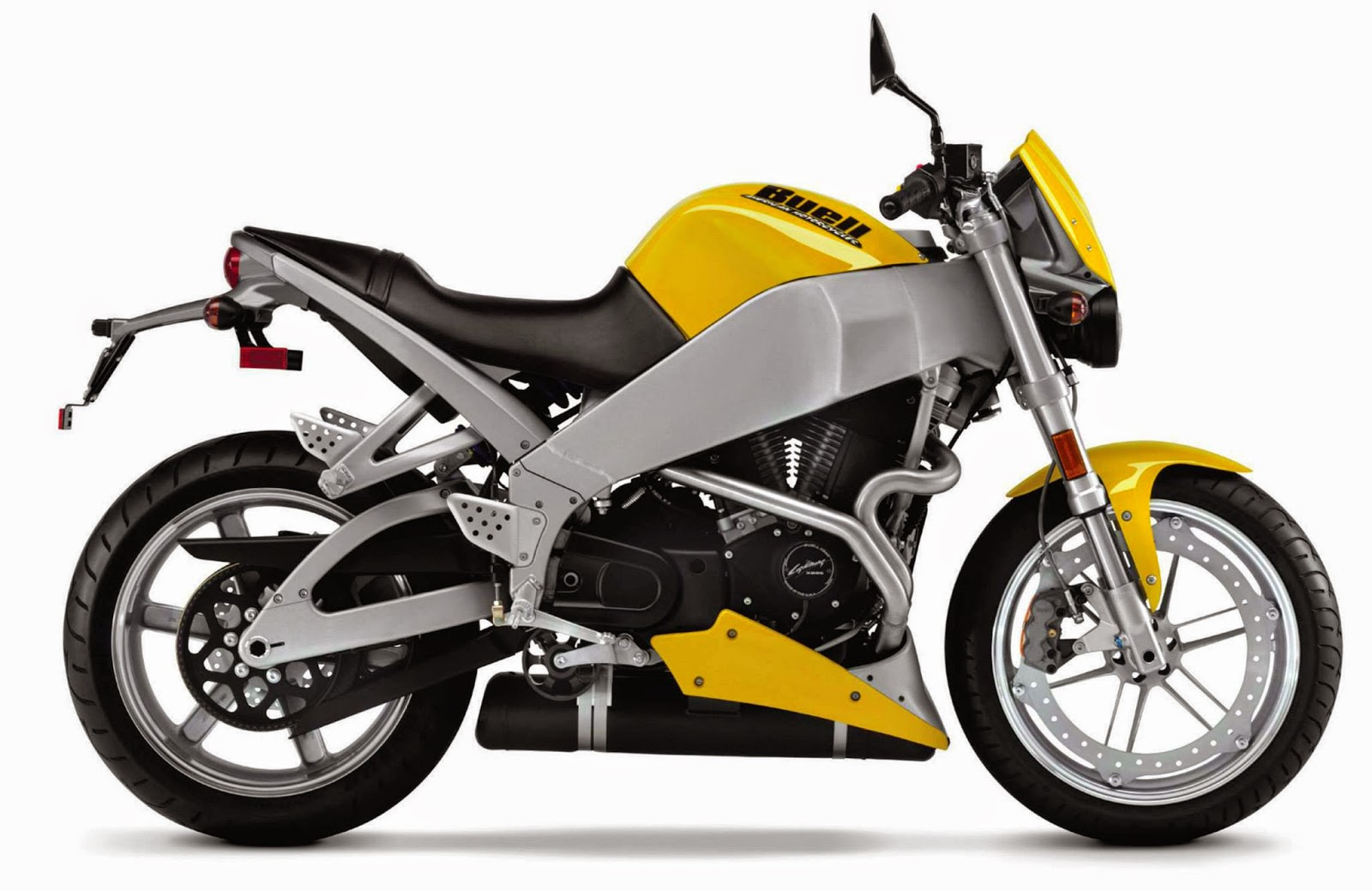### BUELL XB9S LIGHTNING

Model years: 2003 to 2004

Number produced: 4,332

2003 = 4,255

2004 = 77

Displacement: 984cc

Horsepower: 92 @ 7,500 rpm

Torque: 68 ft.lbs @ 5,500 rpm

BUELL XB9SL LIGHTNING LOW

Model years: 2003 to 2004 Number produced: 1,513

2003 = 1,094

2004 = 419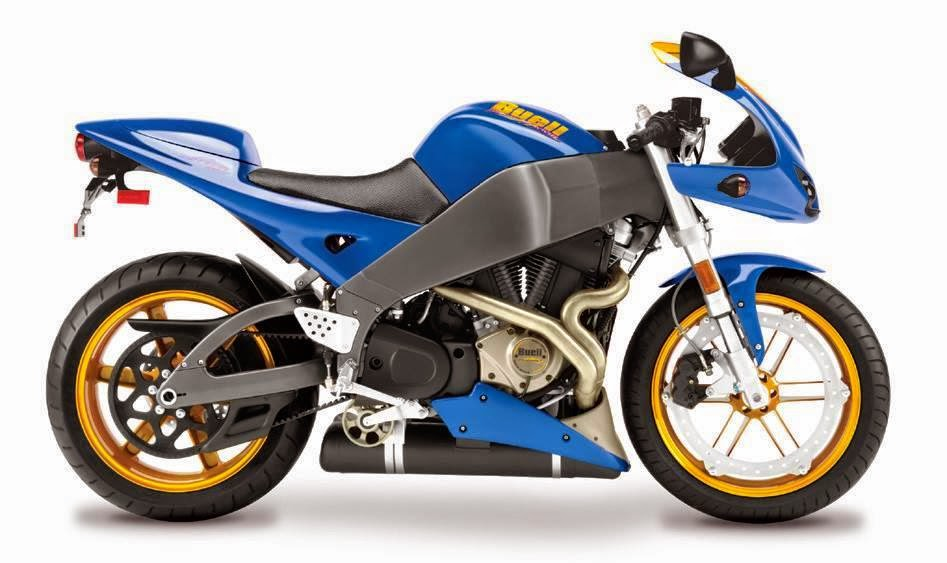### BUELL XB12R FIREBOLT

Model years: 2004 to 2010

Number produced: 10,408

2004 = 3,937

2005 = 1,802

2006 = 1,056

2007 = 1,584

2008 = 801

2009 = 975

2010 = 253

Displacement: 1203cc

Horsepower: 103 @ 6,800 rpm

Torque: 84 ft.lbs @ 6,000 rpm

### BUELL XB12S LIGHTNING

Model years: 2004 to 2008

Number produced: 10,852

2004 = 5,111

2005 = 2,321

2006 = 529

2007 = 1,708

2008 = 1,183

Displacement: 1203cc

Horsepower: 103 @ 6,800 rpm

Torque: 84 ft.lbs @ 6,000 rpm

### BUELL XB12Scg LIGHTNING

Model years: 2005 to 2010

Number produced: 5,561

2005 = 732

2006 = 290

2007 = 1,409

2008 = 1,263
2009 = 1,508
2010 = 357

Displacement: 1203cc

Horsepower: 103 @ 6,800 rpm

Torque: 84 ft.lbs @ 6,000 rpm

### BUELL XB9SX LIGHTNING CITY X

Model years: 2005 to 2010

Number produced: 9,632
2005 = 3,095

2006 = 2,296

2007 = 1,427

2008 = 834
2009 = 1,699
2010 = 281

Displacement: 984cc

Horsepower: 92 @ 7,500 rpm

Torque: 70 ft.lbs @ 5,500 rpm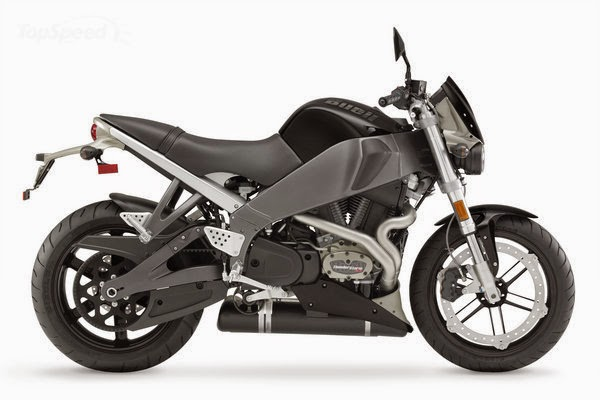### BUELL XB12Ss LIGHTNING LONG

Model years: 2006 to 2010

Number produced: 7,013

2006 = 2,205

2007 = 1,724

2008 = 1,263
2009 = 909
2010 = 283

Displacement: 1203cc

Horsepower: 103 @ 6,800 rpm

Torque: 84 ft.lbs @ 6,000 rpm

### BUELL XBRR

Model year 2007

Number produced: 56

Displacement: 1338cc

Horsepower: 150 @ 8,000 rpm

Torque: 100 ft.lbs @ 6,400 rpm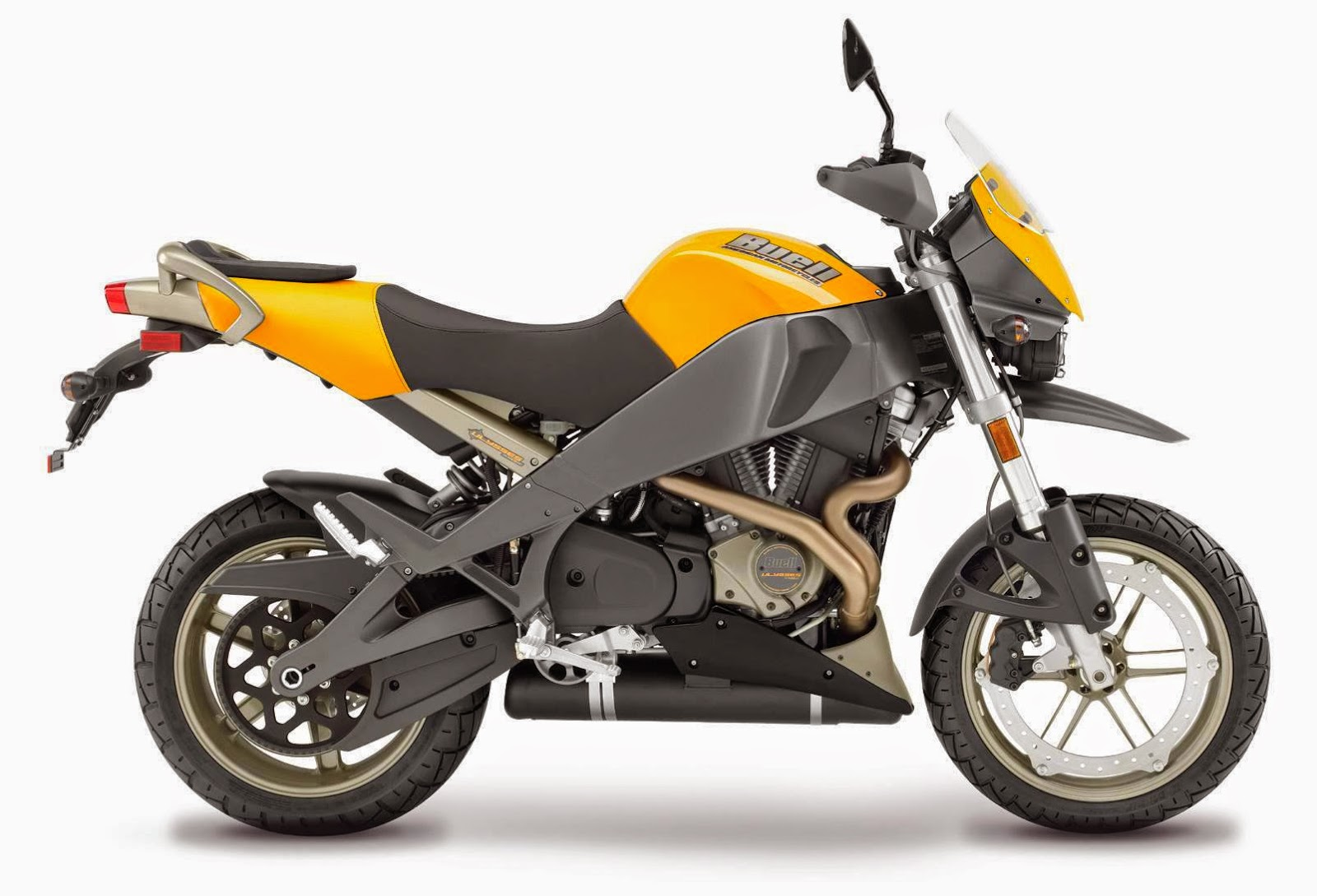### BUELL XB12X ULYSSES

Model years: 2006 to 2010

Number produced: 7,432

2006 = 4,279

2007 = 1,396

2008 = 975
2009 = 686
2010 = 96

Displacement: 1203cc

Horsepower: 103 @ 6,800 rpm

Torque: 84 ft.lbs @ 6,000 rpm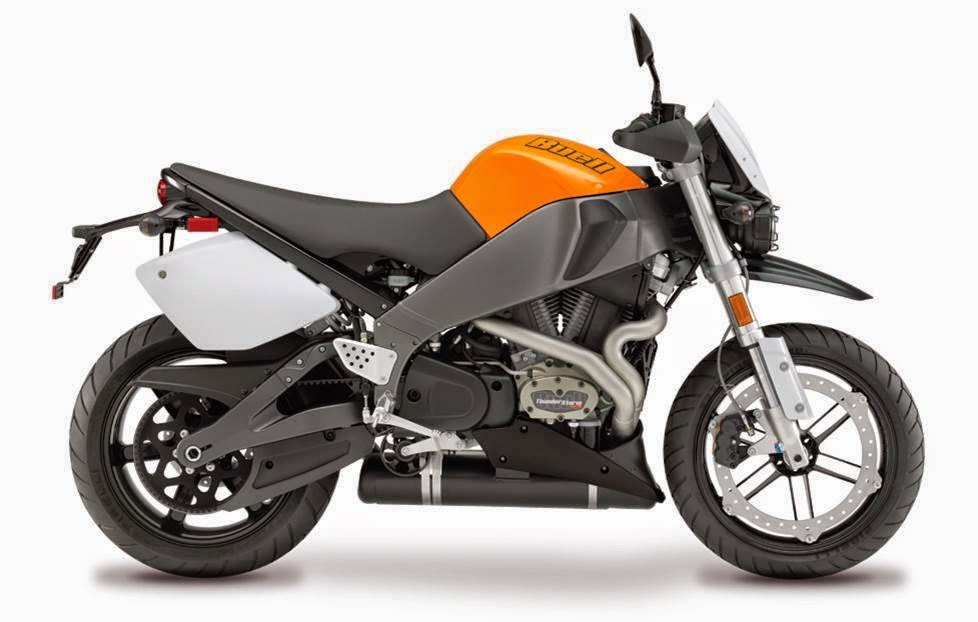### BUELL XB12STT LIGHTNING SUPER TT

Model years: 2007 to 2008

Number produced: 1,755

2007 = 1,649

2008 = 106
Displacement: 1203cc

Horsepower: 103 @ 6,800 rpm

Torque: 84 ft.lbs @ 6,000 rpm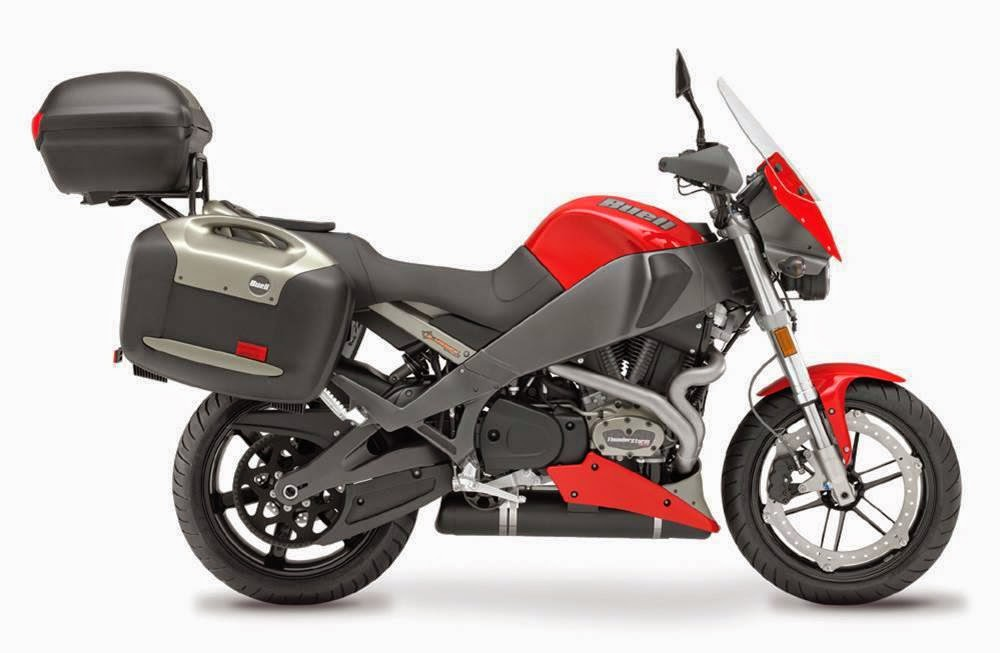### BUELL XB12XT ULYSSES

Model years: 2008 to 2010

Number produced: 1,911

2008 = 869

2009 = 831
2010 = 211

Displacement: 1203cc

Horsepower: 103 @ 6,800 rpm

Torque: 84 ft.lbs @ 6,000 rpm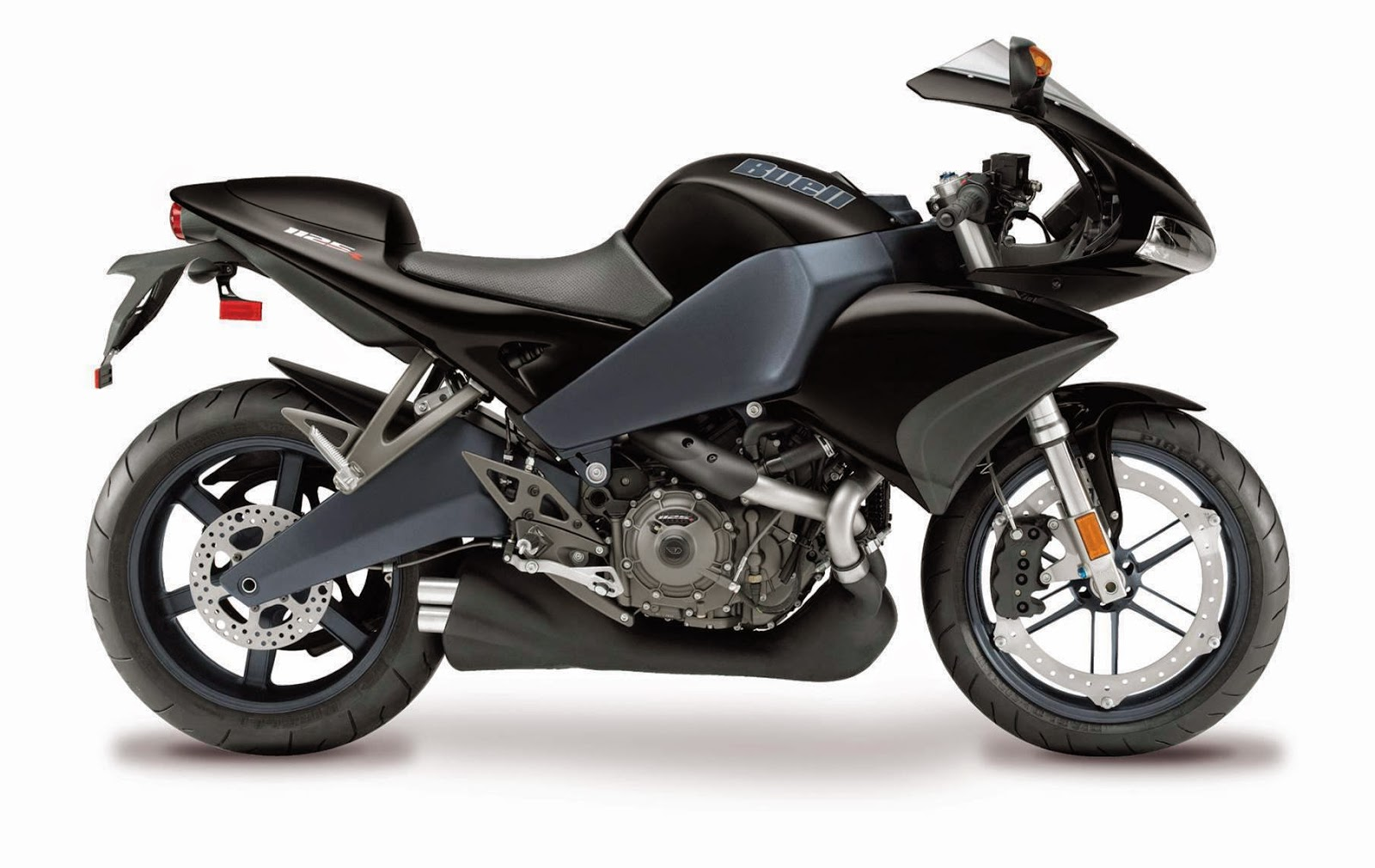### Model years: 2008 to 2010

Number produced: 5,836

2008 = 3,927

2009 = 1,814
2010 = 95

Displacement: 1125cc

Horsepower: 146 @ 9,800 rpm

Torque: 82 ft.lbs @ 8,000 rpm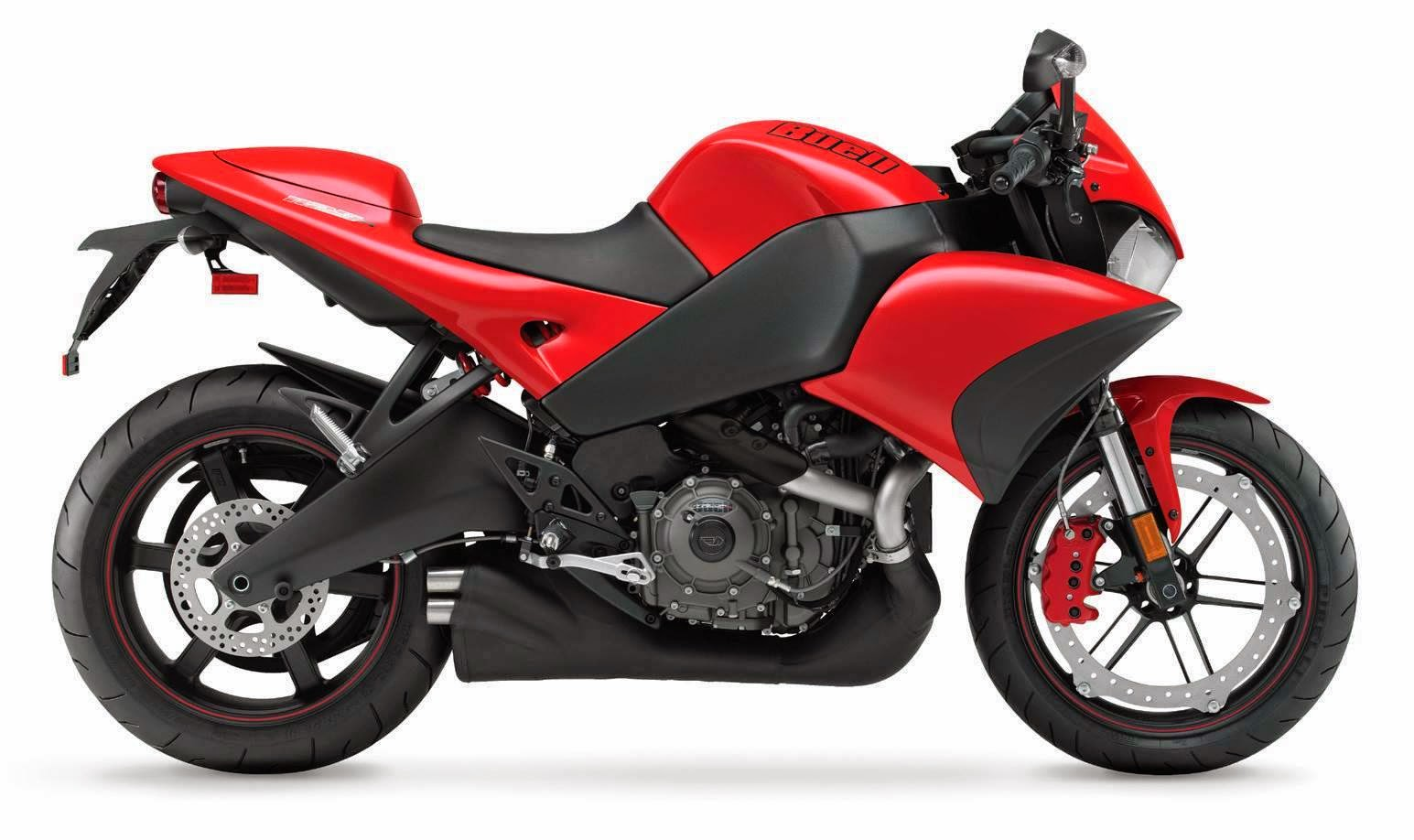### BUELL 1125CR

Model years: 2009 to 2010

Number produced: 3,099

2009 = 2,868

2010 = 231

Displacement: 1125cc

Horsepower: 146 @ 9,800 rpm

Torque: 82 ft.lbs @ 8,000 rpm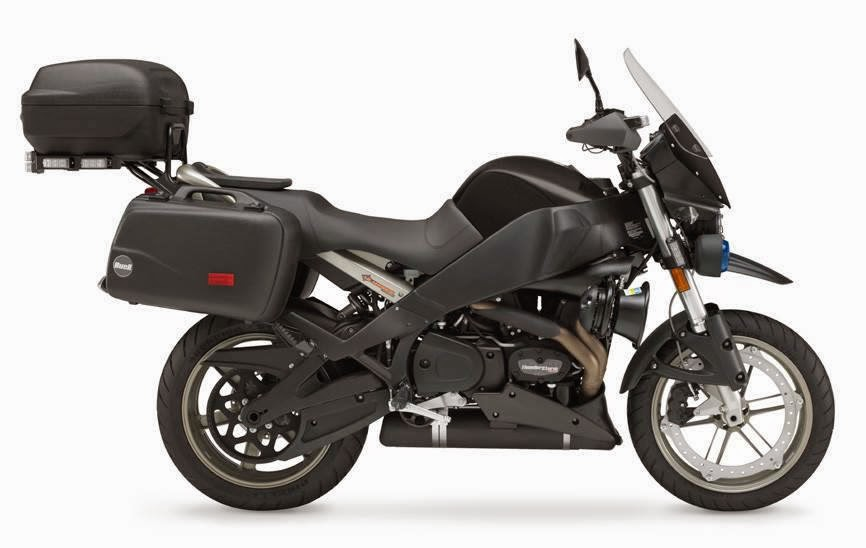### BUELL XB12XP POLICE

Model years: 2009 to 2010

Number produced: 137

2009 = 122

2010 = 15

Displacement: 1203cc

Horsepower: 103 @ 6,800 rpm

Torque: 84 ft.lbs @ 6,000 rpm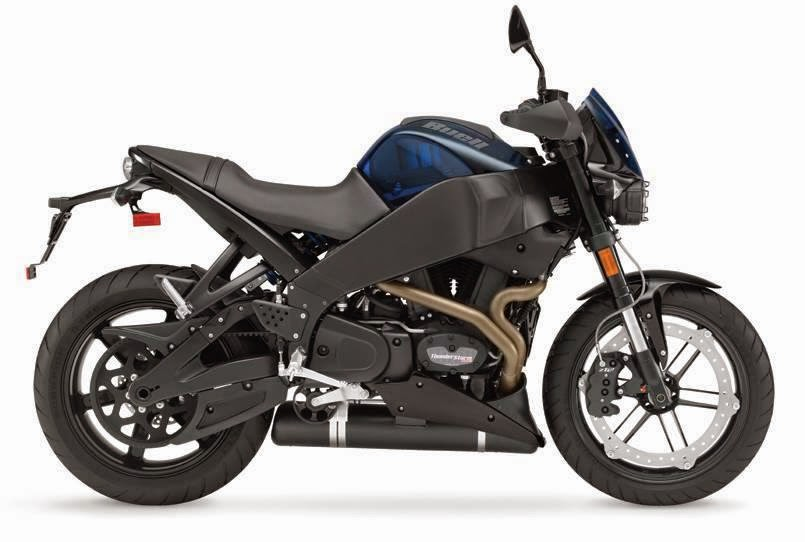### BUELL XB12SX LIGHTNING CITY X

Model years: 2010

Number produced: 860

Displacement: 1203cc

Horsepower: 103 @ 6,800 rpm

Torque: 84 ft.lbs @ 6,000 rpm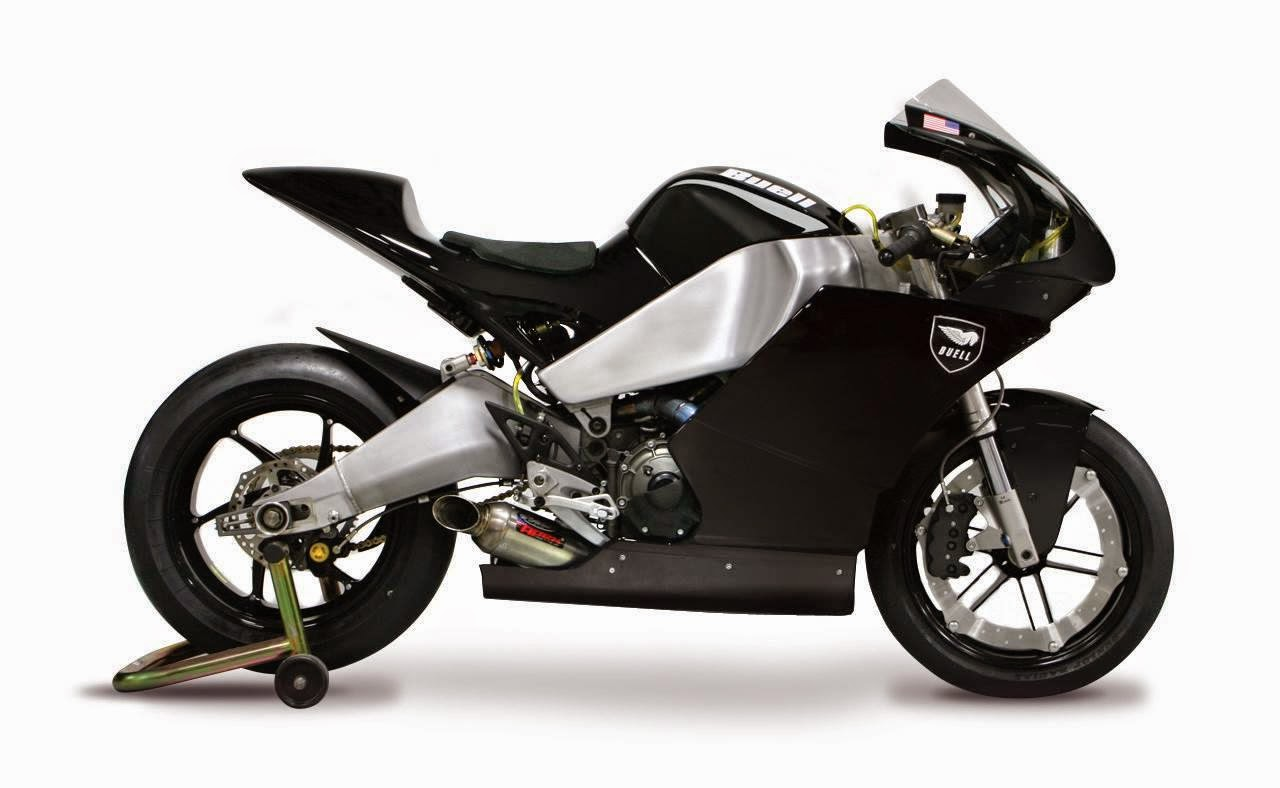### BUELL 1125RR

Model years: 2010

Number produced: 3

Displacement: 1125cc

Horsepower: 171 @ 11,500 rpm

Torque: 86 ft.lbs @ 10,000 rpm